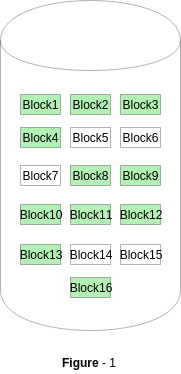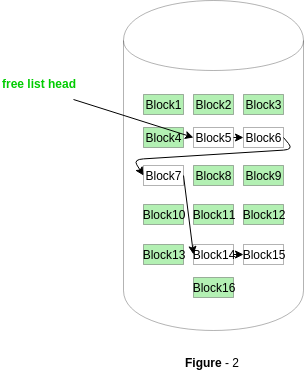Related Articles
Free space management in Operating System
• Difficulty Level : Easy
• Last Updated : 14 Aug, 2019

The system keeps tracks of the free disk blocks for allocating space to files when they are created. Also, to reuse the space released from deleting the files, free space management becomes crucial. The system maintains a free space list which keeps track of the disk blocks that are not allocated to some file or directory. The free space list can be implemented mainly as:

1. Bitmap or Bit vector –
A Bitmap or Bit Vector is series or collection of bits where each bit corresponds to a disk block. The bit can take two values: 0 and 1: 0 indicates that the block is allocated and 1 indicates a free block.
The given instance of disk blocks on the disk in Figure 1 (where green blocks are allocated) can be represented by a bitmap of 16 bits as: 0000111000000110.Advantages –

• Simple to understand.
• Finding the first free block is efficient. It requires scanning the words (a group of 8 bits) in a bitmap for a non-zero word. (A 0-valued word has all bits 0). The first free block is then found by scanning for the first 1 bit in the non-zero word.

The block number can be calculated as:
(number of bits per word) *(number of 0-values words) + offset of bit first bit 1 in the non-zero word .

For the Figure-1, we scan the bitmap sequentially for the first non-zero word.
The first group of 8 bits (00001110) constitute a non-zero word since all bits are not 0. After the non-0 word is found, we look for the first 1 bit. This is the 5th bit of the non-zero word. So, offset = 5.
Therefore, the first free block number = 8*0+5 = 5.

2. Linked List –
In this approach, the free disk blocks are linked together i.e. a free block contains a pointer to the next free block. The block number of the very first disk block is stored at a separate location on disk and is also cached in memory.In Figure-2, the free space list head points to Block 5 which points to Block 6, the next free block and so on. The last free block would contain a null pointer indicating the end of free list.
A drawback of this method is the I/O required for free space list traversal.

3. Grouping –
This approach stores the address of the free blocks in the first free block. The first free block stores the address of some, say n free blocks. Out of these n blocks, the first n-1 blocks are actually free and the last block contains the address of next free n blocks.
An advantage of this approach is that the addresses of a group of free disk blocks can be found easily.
4. Counting –
This approach stores the address of the first free disk block and a number n of free contiguous disk blocks that follow the first block.
Every entry in the list would contain:
1. Address of first free disk block
2. A number n

For example, in Figure-1, the first entry of the free space list would be: ([Address of Block 5], 2), because 2 contiguous free blocks follow block 5.

Attention reader! Don’t stop learning now. Get hold of all the important CS Theory concepts for SDE interviews with the CS Theory Course at a student-friendly price and become industry ready.

My Personal Notes arrow_drop_up